Trial ends in

# Determining Rate Laws and the Order of Reaction

### Overview

Source: Laboratory of Dr. Neal Abrams — SUNY College of Environmental Science and Forestry

All chemical reactions have a specific rate defining the progress of reactants going to products. This rate can be influenced by temperature, concentration, and the physical properties of the reactants. The rate also includes the intermediates and transition states that are formed but are neither the reactant nor the product. The rate law defines the role of each reactant in a reaction and can be used to mathematically model the time required for a reaction to proceed. The general form of a rate equation is shown below:where A and B are concentrations of different molecular species, m and n are reaction orders, and k is the rate constant. The rate of nearly every reaction changes over time as reactants are depleted, making effective collisions less likely to occur. The rate constant, however, is fixed for any single reaction at a given temperature. The reaction order illustrates the number of molecular species involved in a reaction. It is very important to know the rate law, including rate constant and reaction order, which can only be determined experimentally. In this experiment, we will explore one method for determining the rate law and use it to understand the progress of a chemical reaction.

### Principles

Kinetics and Thermodynamics

All chemical reactions are governed by two factors, kinetics and thermodynamics. The thermodynamic factor is the difference in free energy released during a chemical reaction. This free energy, termed spontaneity, is a complex value arising from the enthalpy (heat) and entropy (disorder) within a chemical reaction. Kinetics refers to the rate of a chemical reaction and how fast the system reaches equilibrium. While kinetics can explain the speed of the reaction, thermodynamics yields information about its energetics. Simply stated, thermodynamics relates to stability and kinetics relates to reactivity.

Determining Rate

Rate is a time-based measurement, meaning it is constantly changing as a reaction proceeds. This can be represented using a differential rate law, expressing the change in concentration over a change in time. Experimentally, the differential rate law is difficult to use, so we can use calculus and represent the rate law as the integrated rate law by integrating the differential rate law. The integrated rate law represents the reaction concentrations at the start of the reaction and at a specified time interval. A table of order, rate law, and integrated rate law is shown below: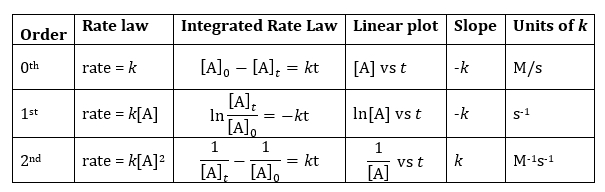Each order explains the dependence of reactant concentration on reaction rate. For example, a zeroth order rate law, rate = k, indicates that the rate is only dependent on the rate constant, not on reactant concentration. This is common in catalytic reactions where the catalyst is a solid and the surface area does not change during the reaction. A 1st order rate law shows that the rate is dependent on the concentration of one reactant, though other reactants may be present. A 2nd order rate law indicates that the rate is dependent on the concentration of two reactants in the reaction. Those reactants can be the same, i.e. rate = k[A]2, or different, rate = k[A][B]. Since the two concentrations are constantly changing, second order rate constants can be difficult to measure in the lab. Regardless of the order, using the integrated rate law simplifies data analysis by allowing for data plotting and applying a linear equation to fit the data. Since only one integrated rate law will fit the data, a reaction's rate constant and reaction order can be immediately identified.

A Kinetics Experiment

Determining the rate law begins with setting up a kinetics experiment for the chemical reaction. A kinetics experiment is carefully controlled so that measurements are made in timed intervals in order to determine the change in concentration of a species over time. That species can either be a reactant (decreasing concentration with time) or a product (increasing concentration with time). If multiple reactants are involved, it is also very important that the concentration of only one reactant changes with time. Increasing the concentration of the other reactants much higher than the reactant being studied makes it appear that the concentration of only one reactant changes during the experiment.

In this experiment, the catalytic decomposition of hydrogen peroxide over a platinum catalyst is explored. Since the platinum is a catalyst, only one species is involved which decomposes into two products according to the reaction below:

2 H2O2(aq) → O2(g) + 2 H2O(l)

Because one of the products, O2, is a gas, the increase in pressure of the system over time can be measured and the Ideal Gas Law (PV = nRT) used to relate pressure to moles. Once that is done for several different concentrations of the reactant, the reaction order and rate law can be solved.

### Procedure

1. Preparing H2O2 Dilutions

1. Stock 3% hydrogen peroxide has a concentration of 0.882 M. Prepare 5 dilutions ranging from 0.882 M to 0.176 M (Table 1). Prepare these solutions volumetrically, but prepare them additively since the solute is very dilute and volumes of water are additive.
2. Place the solutions in a constant temperature water bath or leave them on the bench top to equilibrate at room temperature. A temperature range of 20–25 °C (293–298 K) is good for this reaction.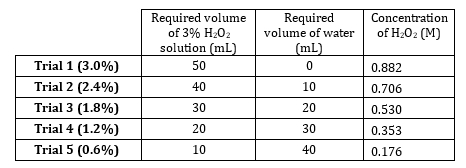Table 1. H2O2 solutions used.

2. Preparing the Reaction Vessel

1. To determine the volume of the reaction vessel, fill a large test tube to the top with water and insert a 1-hole rubber stopper into the test tube until tight and water pushes out the sides and through the top.
2. Remove the stopper and pour the water into a graduated cylinder to determine the exact volume of the water. This is the total volume of the reaction vessel (test tube).

3. Measuring Oxygen Evolution

1. Replace the water with 50 mL of the first hydrogen peroxide solution and place it back into the water bath. Once equilibrated, add the platinum-coated reaction disc and seal the system with a stopper connected to a gas pressure sensor. These discs are commonly used in contact lens cleaning systems.
2. Once the pressure sensor is setup to acquire data at 2 points/s, run the experiment for 120 s. The Vernier gas pressure sensor, GPS-BTA, is recommended for this experiment.
3. Bubbles should be observed as the peroxide is decomposed to oxygen gas and water. Release the pressure, dispose of the solution, rinse, and replace the solution with the next hydrogen peroxide solution. Repeat the gas pressure measurement until all solutions are tested.

4. Data Analysis

1. Transfer all data files of pressure versus time to a spreadsheet program.
2. Determining initial rates -assume that the concentration of hydrogen peroxide has not changed much during the short timeframe of the experiment. The data represent the initial linear region of the kinetics experiment.
1. Determine slope by plotting pressure versus time and using a slope formula or linear regression. Plot the pressure in any common unit.
2. The slope is the initial rate in units of pressureO2/s.
3. Determining Reaction Order
1. Because pressure of evolved O2 is directly proportional to the moles of decomposed H2O2, plotting the ln(initial rate) vs. ln[H2O2]0 yields a slope equivalent to the order of the reaction. The initial concentration of hydrogen peroxide, [H2O2]0, is what was used in each of the trials.
1. The equation for the rate law is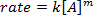. Taking the natural logarithm (ln) of the equation produces a linear equation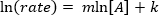, where m, the slope, is the order of the reaction.
1. Determining the Rate Constant, k
1. For each trial, convert the rate, PO2/s, into units of atm/s if the rate is in a different unit such as torr/s.
2. Because bubbles were evolved in aqueous solution, subtract the vapor pressure of water from the system pressure for each trial. The new rate reflects only the pressure due to oxygen evolution.
3. Apply the Ideal Gas law to convert the rate from atm/s into moles/s in each trial.
1. Rearrange PV = nRT to n = PV/RT. The s-1 unit remains unchanged. The volume is equivalent to the test tube volume minus the solution volume (50 mL).
4. Use the balanced chemical reaction to convert from moles of oxygen produced to moles of hydrogen peroxide decomposed in each trial.
5. Divide the moles of H2O2 by the volume of the solution, 0.050 L, to yield the molarity of H2O2 decomposed per second, [H2O2]/s.
6. Because this experiment follows first-order kinetics, divide the rate, [H2O2]/s, by the original solution concentration for each trial, [H2O2]0, to yield a rate constant, k. This solution for the rate constant would vary slightly based on the order of the reaction previously determined.
7. Average the rate constants for each trial together since the temperature is constant.

All chemical reactions have a specific rate, which defines how quickly the reactants turn into products.

Chemical reactions are governed by two factors: kinetics and thermodynamics. Thermodynamic factors account for whether or not a reaction will occur, and if it absorbs or gives off energy during the process.

Kinetics refers to the rate of a chemical reaction, and how fast the system reaches equilibrium. The kinetics of a reaction is described by the kinetic rate law, which defines the rate based on a reaction rate constant, the concentration of components, and the order of the reaction.

This video will introduce reaction kinetics using the rate law equation, and will demonstrate how to determine the rate law for a certain reaction in the laboratory.

For a general reaction, the reaction rate equals the rate constant times the concentrations of the reactants, each raised to a reaction order. The rate constant, k, is fixed for a reaction at a given temperature.

The reaction orders are independent of the stoichiometric coefficients. Instead they are dependent on the reaction mechanism, and illustrate how the rate is related to the concentration of reactants. For example, if the reaction rate is unchanged when the concentration of "A" doubles, then the reaction does not depend on concentration, and the order is zero.

If the rate doubles when the concentration of reactant "A" doubles, then the reaction is first order with respect to "A." The same behavior is true for reactant "B". The overall order of a reaction is the sum of the individual reaction orders for each reactant.

During a reaction, the concentration of reactants changes with time. Note that that the basic rate equation does not include time as a variable, and can only relate rate and concentration at a specific time point. However, the rate changes as the reaction proceeds and as reactants are depleted. Using a differential rate law can relate the change in concentration to time.

The rate law for a reaction must be determined experimentally, where a chemical reaction is carefully controlled at a constant temperature, and the concentration of the reactants or products measured at specific time intervals. Since concentration measurements are made at discrete time points, the differential rate law is difficult to correlate to experimental data.

Integrating the differential rate law results in a simpler equation, called the integrated rate law. The integrated rate law compares the reactant concentrations at the start of the reaction and at a specified time.

The integrated rate law equation varies depending on the order of the reaction. These equations can take the linear form y=mx+b. Thus, a plot of concentration vs time yields a linear plot for a zero order equation, a plot of the natural log of concentration vs time yields a linear plot for a first order equation, and so on. By fitting experimental data to these equations, the order of a reaction can be easily determined. The rate constant, k, can then be determined using the slope of the line. Finally, the units of k vary depending on the order of the reaction. For a zero order reaction, the units are moles per liter per second, for a first order reaction, the units are inverse seconds and for a second order reaction the units are liter per mole per second.

Now that the basics of kinetic rate laws have been explained, lets take a look at how to experimentally determine the rate law for the decomposition of hydrogen peroxide to water and oxygen.

In this experiment, the catalytic decomposition of hydrogen peroxide over a platinum catalyst is explored.

First, prepare 5 dilutions of hydrogen peroxide, as shown in the table. In this case, concentrations range from 0.882 – 0.176 M, using a 3% or 0.882 M stock solution. Allow the solutions to equilibrate to room temperature.

Next, prepare the reaction vessel using a test tube. First determine the volume by filling a large test tube to the top with water. Then, insert a 1-hole rubber stopper until it is tight and water pushes out of the hole through the top.

Remove the stopper, and pour the water into a graduated cylinder to measure the exact volume. This is the volume of the reaction vessel.

Next, pour 50 mL of the first hydrogen peroxide solution into the test tube, and then place the tube in the 25 °C water bath. Once equilibrated, add a platinum coated reaction disc, and seal the system with a stopper connected to a gas pressure sensor.

Since one of the products is oxygen gas, the increase of pressure in the system is used to measure the increase in oxygen. Set up the pressure sensor to acquire data at 2 points per s, then run the experiment for 120 s. Bubbles should be visible as the peroxide decomposes to oxygen gas and water.

When the reaction period is over, release the pressure and dispose of the peroxide solution. Rinse the tube, then fill the tube with the next hydrogen peroxide solution. Repeat the gas pressure measurement for all solutions.

Plot the pressure versus time data for each solution. The pressure of evolved oxygen is directly proportional to the moles of oxygen formed according to the ideal gas law. Following the chemical reaction, the moles of oxygen formed can be used to calculate the moles of decomposed hydrogen peroxide. First, assume that the concentration of hydrogen peroxide did not change significantly during the short duration of the experiment. Thus, the plotted data represents only the initial region of the kinetics experiment.

Determine the slope of each data set using a linear regression. The slope is equal to the initial reaction rate in units of pressure of oxygen per second.

Next, plot of the natural log of the initial reaction rate vs the natural log of initial peroxide concentration. The slope is equal to the reaction order, m, and is approximately equal to one. Therefore, the reaction is first order.

The rate for each trial is in units of pressure in Torr, per second. To determine the rate constant, first convert the rate to units of atmospheres per second. Because the bubbles evolved in the aqueous solution, subtract the vapor pressure of water from the system pressure for each trial. The new rate then only reflects the pressure due to oxygen evolution.

Apply the ideal gas law to convert the rate from atmospheres per se to moles per s for each trial. Two times the moles of oxygen produced are equal to the moles of hydrogen peroxide decomposed, according to the chemical reaction stoichiometry. Then, use the reaction volume to convert the units of the rate to molarity per second.

Determine the rate constants for each trial by dividing the rate in molarity per s by the initial concentration. In this experiment, the average rate constant, k, is approximately 1.48 x 10-4 per s. The reaction is first order, known from the natural log - natural log plot shown earlier. Therefore, the rate law can be written as shown.

Now that you've looked at how to determine the rate law for a chemical reaction, lets look at some areas where this concept is applied.

Chemical reactions are used in the synthesis of compounds and materials used in a wide range of scientific applications. It is important to understand the reaction rate in these synthesis steps, in order to control the progress of a reaction.

For example, the synthesis of cadmium selenide nanocrystals and nanorods proceeds through a series of chemical reactions. Each reaction has its own discrete reaction rate, and therefore synthesis step is carefully controlled based on the knowledge speed of the reaction; some slow and some very quick.

The reaction rate law can also be used to describe radioactive decay, and determine the half-life of radioactive material. Half-life refers to the amount of time required for the concentration of a material to fall to half of its initial concentration.

Radioactivity follows first order kinetics, meaning that the time required for radioactive material to decay to a safe level can be very well characterized, enabling proper transport and storage of radioactive material and radioactive waste.

Similar to radioactive materials, drugs also have a half-life and degrade in the body. For example, some drugs have high rate constants, meaning that they degrade quickly, and must be taken frequently. Knowledge of this degradation rate enables the determination of the appropriate dosage, usage and delivery method.

You've just watched JoVE's introduction to reaction rates. You should now understand the different orders of chemical reactions, how they relate to chemical reaction rates, and how to determine the rate law for a given chemical reaction in the laboratory.

Thanks for watching!

### Results

Oxygen Evolution Data and Initial Rates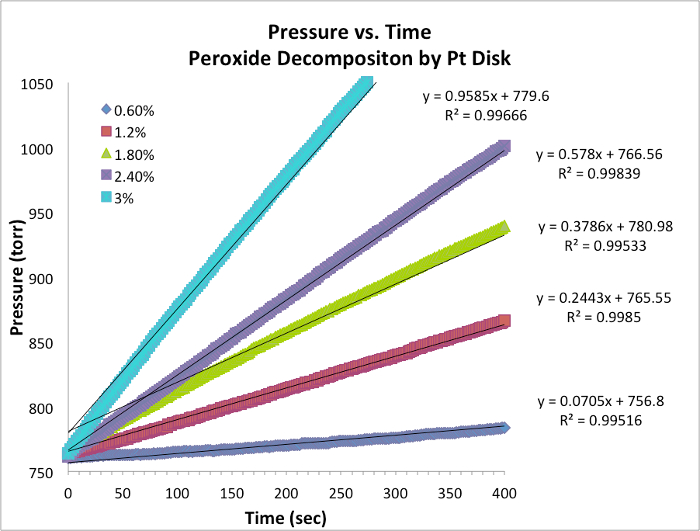Figure 1. Pressure vs. time data for each trial at constant temperature. The slope is equivalent to the instantaneous rate of the reaction.

Reaction Order

1. Data for five trials and graph with slope is tabulated below (Table 2, Figure 2). The slope of 0.9353 ≈ 1, is an integer value of the reaction order.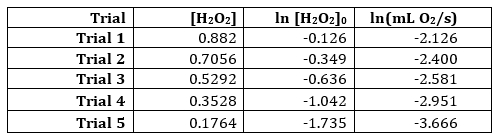Table 2. Results from 5 H2O2 trials.Figure 2. Natural log plot of concentration vs. rate. The slope is equivalent to the order of the reaction.

Rate Constant, k

1. Calculate the rate in mol O2/s using the Ideal Gas law by first subtracting out the vapor pressure of water at 294 K (temperature of the kinetics experiment), 18.7 torr.
2. Calculate the rate constant by dividing the rate, [H2O2]/s by the initial concentration of [H2O2]0.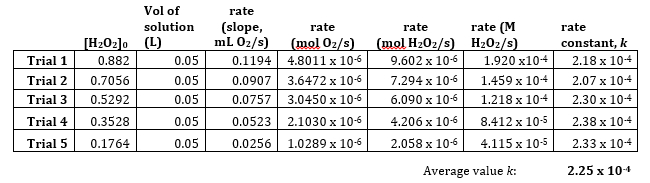Table 3. Calculation of the rate constant, k.

1. The average rate constant, 2.25 x 10-4 s-1 indicates the reaction is first order based on the units. The rate law could be rewritten as: rate = 2.25 x 10-4 s-1[H2O2]0

### Applications and SummaryX